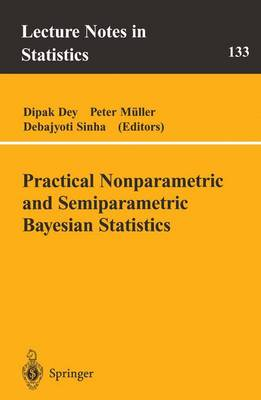•Stochastic Processes and Orthogonal Polynomials - Lecture Notes in Statistics 146 (Paperback)

(author)
£99.99
Paperback 184 Pages / Published: 27/04/2000
• We can order this

Usually dispatched within 3 weeks

It has been known for a long time that there is a close connection between stochastic processes and orthogonal polynomials. For example, N. Wiener  and K. Ito  knew that Hermite polynomials play an important role in the integration theory with respect to Brownian motion. In the 1950s D. G. Kendall , W. Ledermann and G. E. H. Reuter  , and S. Kar- lin and J. L. McGregor  established another important connection. They expressed the transition probabilities of a birth and death process by means of a spectral representation, the so-called Karlin-McGregor representation, in terms of orthogonal polynomials. In the following years these relation- ships were developed further. Many birth and death models were related to specific orthogonal polynomials. H. Ogura , in 1972, and D. D. En- gel , in 1982, found an integral relation between the Poisson process and the Charlier polynomials. Some people clearly felt the potential im- portance of orthogonal polynomials in probability theory. For example, P. Diaconis and S. Zabell  related Stein equations for some well-known distributions, including Pearson's class, with the corresponding orthogonal polynomials. The most important orthogonal polynomials are brought together in the so-called Askey scheme of orthogonal polynomials. This scheme classifies the hypergeometric orthogonal polynomials that satisfy some type of differ- ential or difference equation and stresses the limit relations between them.

Publisher: Springer-Verlag New York Inc.
ISBN: 9780387950150
Number of pages: 184
Weight: 590 g
Dimensions: 235 x 155 x 9 mm
Edition: 2000 ed.